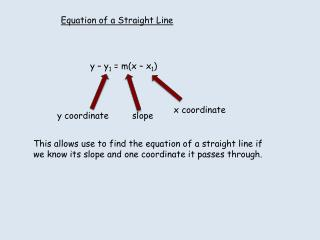DownloadDownload Presentationy – y 1 = m(x – x 1 )

y – y 1 = m(x – x 1 )

Download Presentationy – y 1 = m(x – x 1 )

- - - - - - - - - - - - - - - - - - - - - - - - - - - E N D - - - - - - - - - - - - - - - - - - - - - - - - - - -
Presentation Transcript

1. Equation of a Straight Line y – y1 = m(x – x1) x coordinate y coordinate slope This allows use to find the equation of a straight line if we know its slope and one coordinate it passes through.

2. Find the equation of the line (a) passing through (-2 , 3) with slope = 3 (b) Passing through (4 , 7) with slope = 2/3 y – y1 = m(x – x1) y – 3 = 3(x - -2) y – 3 = 3x + 6 y = 3x + 9 y – y1 = m(x – x1) y – 7 = 2/3(x – 4) 3y – 21 = 2x - 8 3y = 2x + 13

3. Find the equation of the line 1.passing through (2 , 1) with slope = 2 2. Passing through (3 , 2) with slope = 3 3. Passing through (-4 , 2) with slope = 5 4. Passing through (-2 , -3) with slope = -3 5. Passing through (5, 3) with slope = 2/5

4. Find the equation of a straight line parallel to 2y = 4x + 5 which passes through (-1 , 3) 2y = 4x + 5 y – y1 = m(x – x1) y = 2x + 5/3 y = 2x + 5 y – 3 = 2(x - -1) y – 3 = 2x + 2

5. Find the equation of a straight line perpendicular to 3y = x - 2 which passes through (4 , 5) 3y = x - 2 y – y1 = m(x – x1) y = x/3 -2/3 y + 3x = 17 y – 5 = -3(x - 4) m = 1/3 y – 5 = -3x + 12 Perp = -3

6. 1. Find the equation of a straight line parallel to y = 2x + 5 which passes through (4 , 5) 2. Find the equation of a straight line parallel to 2y + 6x = 18 which passes through (2 , -4) 3. Find the equation of a straight line parallel to 3y = 4x - 2 which passes through (3 , 2) 4. Find the equation of a straight line perpendicular to y = 2x + 3 which passes through (1 , 3)

7. Find the equation of the line passing through the points (4 , 5) and (-2, 3). 3 - 5 m = = -2/-6 = 1/3 -2 - 4 y – 5 = 1/3(x – 4) 3y – 15 = x - 4 3y = x + 11

8. Find the equation of the line passing through the points (2 , 3) and (8 , 6) (-3, 1) and (7 , 6)